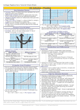How to Learn in 24 Hours?

 Need Help? M-F: 9am-5pm(PST): Toll-Free: (877) RAPID-10 or 1-877-727-4310 24/7 Online Technical Support: The Rapid Support Center vip@rapidlearningcenter.com Secure Online Order:Need Proof? Testimonials by Our Users

 Rapid Learning Courses: MCAT in 24 Hours (2021-22) USMLE in 24 Hours (Boards) Chemistry in 24 Hours Biology in 24 Hours Physics in 24 Hours Mathematics in 24 Hours Psychology in 24 Hours SAT in 24 Hours ACT in 24 Hours AP in 24 Hours CLEP in 24 Hours DAT in 24 Hours (Dental) OAT in 24 Hours (Optometry) PCAT in 24 Hours (Pharmacy) Nursing Entrance Exams Certification in 24 Hours eBook - Survival Kits Audiobooks (MP3)

 Tell-A-Friend: Have friends taking science and math courses too? Tell them about our rapid learning system.Home »  Mathematics »  College Algebra

Calculus Preview

 Topic Review on "Title": Calculus:    Calculus is a branch of mathematics that addresses the use of limits as tools to solve problems. Differential Calculus: Differential Calculus is a branch of mathematics that uses limits to develop derivatives and differential equations. Integral Calculus: Integral Calculus is a branch of mathematics that uses limits to find integrals.

Rapid Study Kit for "Title":
 Flash Movie Flash Game Flash Card Core Concept Tutorial Problem Solving Drill Review Cheat Sheet"Title" Tutorial Summary : This tutorial specifically describes the basic concepts of calculus. Basic principles of calculus are shown through graphical representation. The tangent line problem is defined in a graph and how it relates to the rate of change. The area of any shape is approximated using rectangles. Differentiation and integration ideals are presented with the use of graphical examples. A summary of what it is expected in a calculus course is described in this tutorial. The differences between calculus and other mathematics courses are given so anyone who is not familiar to the subject becomes comfortable with the subject.

 Tutorial Features: Specific Tutorial Features: • Graphs showing the differences between calculus and other mathematics courses. • Step by step analysis of the different principles that relate to the integration and differentiation of functions in calculus. Series Features: • Concept map showing inter-connections of new concepts in this tutorial and those previously introduced. • Definition slides introduce terms as they are needed. • Visual representation of concepts • Animated examples—worked out step by step • A concise summary is given at the conclusion of the tutorial.

 "Title" Topic List: Getting to know Calculus Definition of Calculus The rate of change The slope of a tangent Calculus and other mathematics courses Similarities and differences between calculus and other mathematics coursesLimits and Derivatives Integration and SeriesCalculus Tips

See all 24 lessons in College Algebra, including concept tutorials, problem drills and cheat sheets:
Teach Yourself College Algebra Visually in 24 Hours